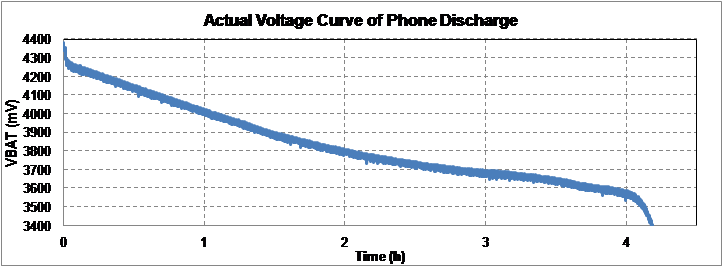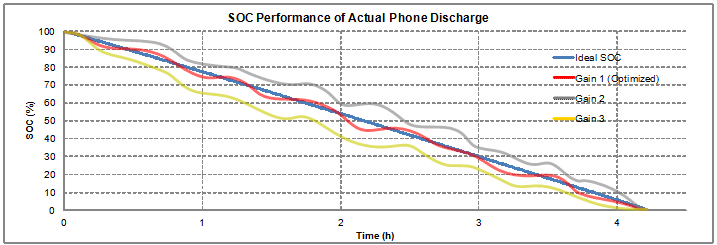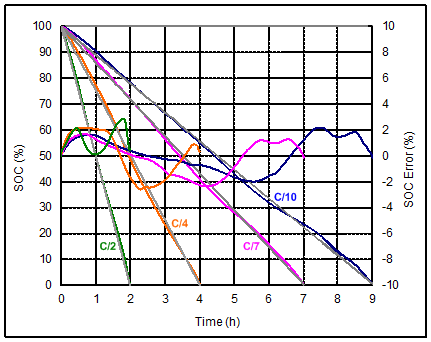# The theory of Lithium charge and discharge & the design of electricity calculation method(3)

The theory of Lithium charge and discharge & the design of electricity calculation method

2.4 Dynamic voltage algorithm electricity meter

The dynamic voltage algorithm coulometer can calculate the state of charge of lithium battery only according to the battery voltage. This method estimates the increment or decrement of the state of charge according to the difference between the battery voltage and the battery open-circuit voltage. Dynamic voltage information can effectively simulate the behavior of lithium battery, and then determine the SOC (%), but this method cannot estimate the battery capacity value (mAh).

Its calculation method is based on the dynamic difference between the battery voltage and the open-circuit voltage, by using the iterative algorithm to calculate each increase or decrease of the state of charge, to estimate the state of charge. Compared with the coulomb metering solution, the dynamic voltage algorithm coulometer will not accumulate errors with time and current. The coulometric coulometer usually has inaccurate estimation of state of charge due to current sensing error and battery self-discharge. Even if the current sensing error is very small, the coulomb counter will continue to accumulate the error, and the accumulated error can only be eliminated after full charging or full discharge.

Dynamic voltage algorithm The electricity meter estimates the state of charge of the battery only from the voltage information; Because it is not estimated by the current information of the battery, it will not accumulate errors. To improve the accuracy of the state of charge, the dynamic voltage algorithm needs to use an actual device to adjust the parameters of an optimized algorithm according to the actual battery voltage curve under the condition of full charge and full discharge.Figure 12. Performance of dynamic voltage algorithm electricity meter and gain optimization

The following is the performance of dynamic voltage algorithm under different discharge rates. It can be seen from the figure that its state of charge accuracy is good. Regardless of the discharge conditions of C/2, C/4, C/7 and C/10, the overall SOC error of this method is less than 3%.Figure 13. State of charge of dynamic voltage algorithm under different discharge rates

The figure below shows the state of charge of the battery under the condition of short charge and short discharge. The error of state of charge is still very small, and the maximum error is only 3%.Figure 14. The state of charge of dynamic voltage algorithm in the case of short charge and short discharge of battery

Compared with coulomb metering coulometer, which usually causes inaccurate state of charge due to current sensing error and battery self-discharge, dynamic voltage algorithm does not accumulate error with time and current, which is a major advantage. Because there is no charge/discharge current information, the dynamic voltage algorithm has poor short-term accuracy and slow response time. In addition, it cannot estimate the full charge capacity. However, it performs well in long-term accuracy because the battery voltage will ultimately directly reflect its state of charge.

Post time: Feb-21-2023
•#### Phone

•